# CHAPTER 2 ContextFree Languages Contents ContextFree Grammars definitions

• Slides: 12CHAPTER 2 Context-Free Languages Contents • Context-Free Grammars • definitions, examples, designing, ambiguity, Chomsky normal form • Pushdown Automata • definitions, examples, equivalence with context-free grammars • Non-Context-Free Languages • the pumping lemma for context-free languages Theory of Computation, Feodor F. Dragan, Kent State University 1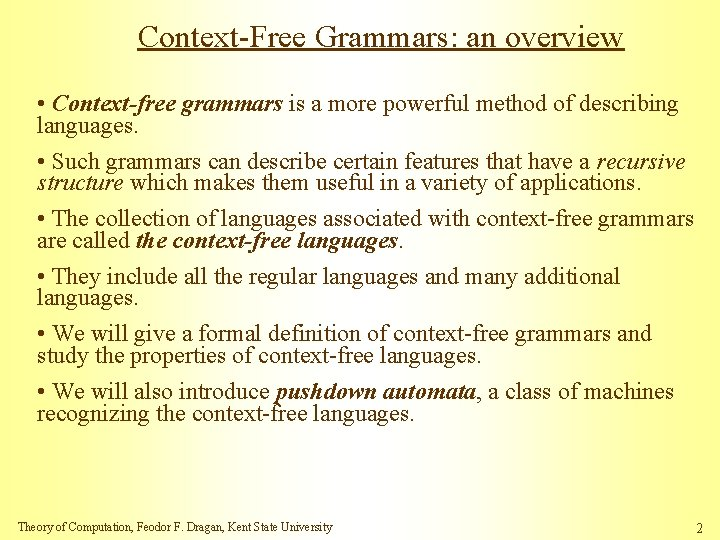Context-Free Grammars: an overview • Context-free grammars is a more powerful method of describing languages. • Such grammars can describe certain features that have a recursive structure which makes them useful in a variety of applications. • The collection of languages associated with context-free grammars are called the context-free languages. • They include all the regular languages and many additional languages. • We will give a formal definition of context-free grammars and study the properties of context-free languages. • We will also introduce pushdown automata, a class of machines recognizing the context-free languages. Theory of Computation, Feodor F. Dragan, Kent State University 2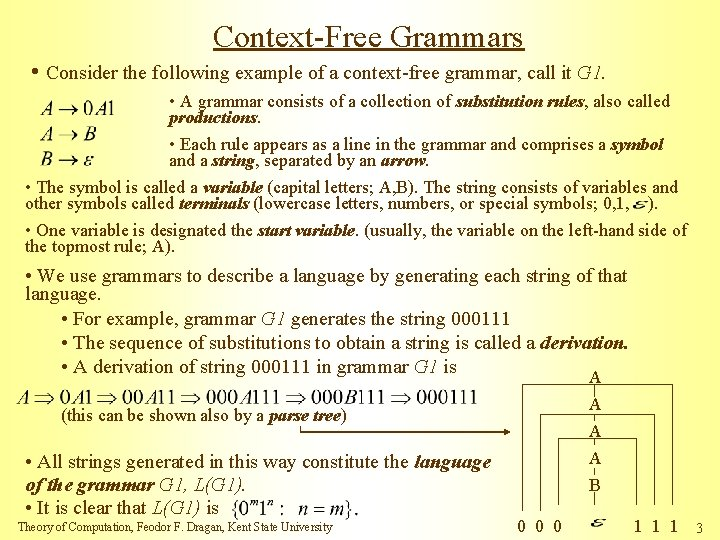Context-Free Grammars • Consider the following example of a context-free grammar, call it G 1. • A grammar consists of a collection of substitution rules, also called productions. • Each rule appears as a line in the grammar and comprises a symbol and a string, separated by an arrow. • The symbol is called a variable (capital letters; A, B). The string consists of variables and other symbols called terminals (lowercase letters, numbers, or special symbols; 0, 1, ). • One variable is designated the start variable. (usually, the variable on the left-hand side of the topmost rule; A). • We use grammars to describe a language by generating each string of that language. • For example, grammar G 1 generates the string 000111 • The sequence of substitutions to obtain a string is called a derivation. • A derivation of string 000111 in grammar G 1 is A A (this can be shown also by a parse tree) • All strings generated in this way constitute the language of the grammar G 1, L(G 1). • It is clear that L(G 1) is Theory of Computation, Feodor F. Dragan, Kent State University A A B 0 0 0 1 1 1 3Context-Free Grammars (cont. ) • Any language that can be generated by some context-free grammar is called a context-free language (CFL) • For convenience when presenting a context-free grammar, we abbreviate several rules with the same left-hand variable, such as A 0 A 1 and A B, into a single line A 0 A 1 | B, using the symbol “|” as an “or”. • Example of a context-free grammar called G 2, which describes a fragment of the English language: • Strings in L(G 2) include the following three examples • Each of these strings has a derivation in grammar G 2. The following is a derivation of the first string on the list Theory of Computation, Feodor F. Dragan, Kent State University 4Formal Definition of a Context-Free Grammar • A context-free grammar is a 4 -tuple , where is a finite set called variables, is a finite set (=alphabet), disjoint from V, called the terminals, is a finite set of rules, with each rule being a variable and a string of variables and terminals, and is the start variable. • If u, v, and w are strings of variables and terminals, and A w is a rule of the grammar, we say that u. Av yields uwv, writing • Write if u=v or if a sequence exists for and • The language of the grammar is • Hence, for G 1: V={A, B}, S=A, and R is the collection of those three rules. for G 2: V={<SENTENCE>, <NOUN-PHRASE>, <VERB-PHRASE>, <PREP-PHRASE>, <CMPLX-NOUN>, <CMPLX-VERB>, <ARTICLE>, <NOUN>, <VERB>, <PREP>}, • Example: G 3=({S}, {(, )}, R, S). The set of rules is L(G 3) is the language of all strings of properly nested parentheses. Theory of Computation, Feodor F. Dragan, Kent State University 5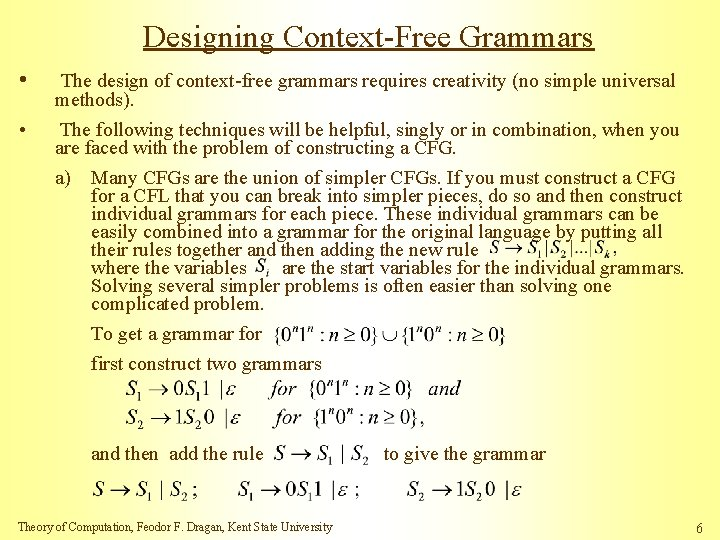Designing Context-Free Grammars • • The design of context-free grammars requires creativity (no simple universal methods). The following techniques will be helpful, singly or in combination, when you are faced with the problem of constructing a CFG. a) Many CFGs are the union of simpler CFGs. If you must construct a CFG for a CFL that you can break into simpler pieces, do so and then construct individual grammars for each piece. These individual grammars can be easily combined into a grammar for the original language by putting all their rules together and then adding the new rule where the variables are the start variables for the individual grammars. Solving several simpler problems is often easier than solving one complicated problem. To get a grammar for first construct two grammars and then add the rule Theory of Computation, Feodor F. Dragan, Kent State University to give the grammar 6Designing Context-Free Grammars (cont. ) b) Constructing a CFG for a language that happens to be regular is easy if you can construct a DFA for that language. You can convert any DFA into an equivalent CFG as follows: • Make a variable for each state of the DFA. • Add rule to the CRG if there is an arc from to with label a. • Add the rule if is an accept state of the DFA. • Make the start variable of the grammar, where is the start state of the machine. Verify on your own that the resulting CFG generates the same language that the DFA recognizes. 0 1 q 0 0 q 1 q 2 1 0, 1 ={w: w contains at least one 1 and an even number of 0 s follow the last 1} • Thus, any regular language is a CFL. Theory of Computation, Feodor F. Dragan, Kent State University 7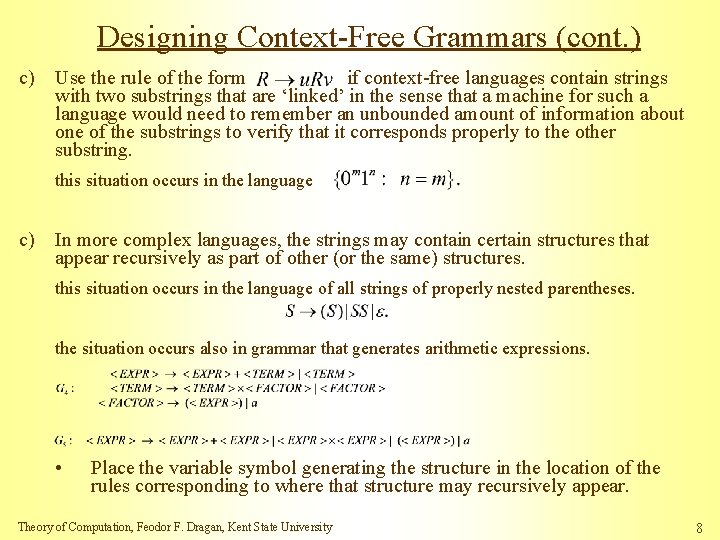Designing Context-Free Grammars (cont. ) c) Use the rule of the form if context-free languages contain strings with two substrings that are ‘linked’ in the sense that a machine for such a language would need to remember an unbounded amount of information about one of the substrings to verify that it corresponds properly to the other substring. this situation occurs in the language c) In more complex languages, the strings may contain certain structures that appear recursively as part of other (or the same) structures. this situation occurs in the language of all strings of properly nested parentheses. the situation occurs also in grammar that generates arithmetic expressions. • Place the variable symbol generating the structure in the location of the rules corresponding to where that structure may recursively appear. Theory of Computation, Feodor F. Dragan, Kent State University 8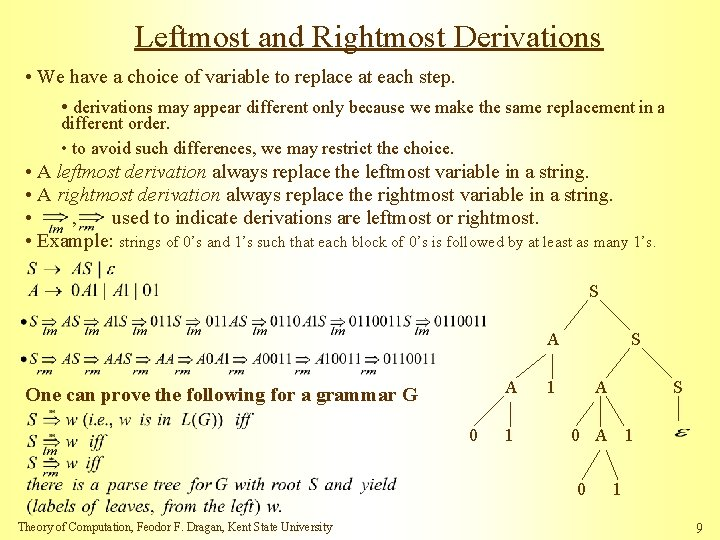Leftmost and Rightmost Derivations • We have a choice of variable to replace at each step. • derivations may appear different only because we make the same replacement in a different order. • to avoid such differences, we may restrict the choice. • A leftmost derivation always replace the leftmost variable in a string. • A rightmost derivation always replace the rightmost variable in a string. • , used to indicate derivations are leftmost or rightmost. • Example: strings of 0’s and 1’s such that each block of 0’s is followed by at least as many 1’s. S A A One can prove the following for a grammar G 0 1 S 1 A 0 A 1 0 Theory of Computation, Feodor F. Dragan, Kent State University S 1 9Ambiguous Grammars • A CFG G is ambiguous if one or more words from L(G) have multiple leftmost derivations from the start variable. • equivalently: multiple rightmost derivations, or multiple parse trees. • Example: consider and the string 00111. { strings of 0’s and 1’s such that each block of 0’s is followed by at least as many 1’s } Inherently Ambiguous Languages • A CFL L is inherently ambiguous if every CFG for L is ambiguous. • such CFLs exist: e. g. , • an inherently ambiguous languages would absolutely unsuitable as a programming language. • The language of our example grammar is not inherently ambiguous, even though the grammar is ambiguous. • Change the grammar to force the extra 1’s to be generated last. Theory of Computation, Feodor F. Dragan, Kent State University 10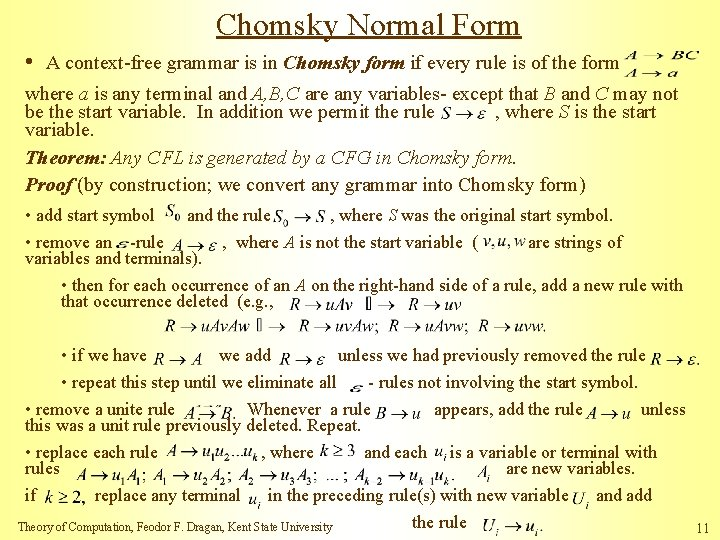Chomsky Normal Form • A context-free grammar is in Chomsky form if every rule is of the form where a is any terminal and A, B, C are any variables- except that B and C may not be the start variable. In addition we permit the rule , where S is the start variable. Theorem: Any CFL is generated by a CFG in Chomsky form. Proof (by construction; we convert any grammar into Chomsky form) • add start symbol and the rule , where S was the original start symbol. • remove an -rule , where A is not the start variable ( are strings of variables and terminals). • then for each occurrence of an A on the right-hand side of a rule, add a new rule with that occurrence deleted (e. g. , • if we have we add unless we had previously removed the rule • repeat this step until we eliminate all - rules not involving the start symbol. • remove a unite rule Whenever a rule appears, add the rule unless this was a unit rule previously deleted. Repeat. • replace each rule , where and each is a variable or terminal with rules are new variables. if replace any terminal in the preceding rule(s) with new variable and add the rule Theory of Computation, Feodor F. Dragan, Kent State University 11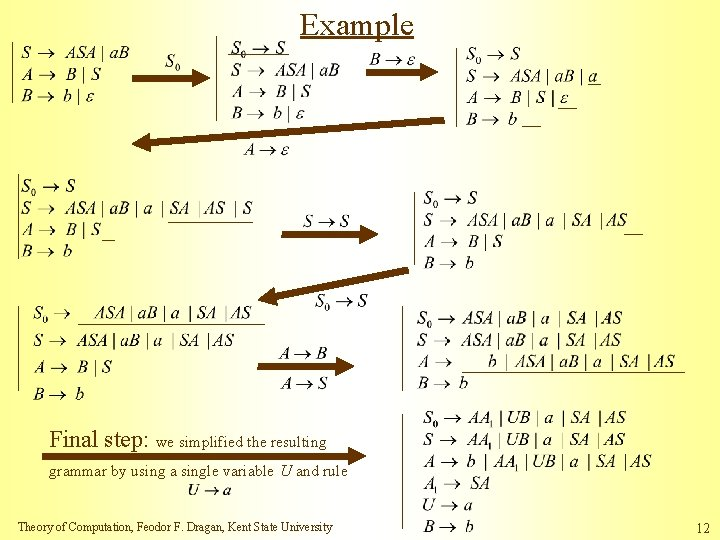Example Final step: we simplified the resulting grammar by using a single variable U and rule Theory of Computation, Feodor F. Dragan, Kent State University 12